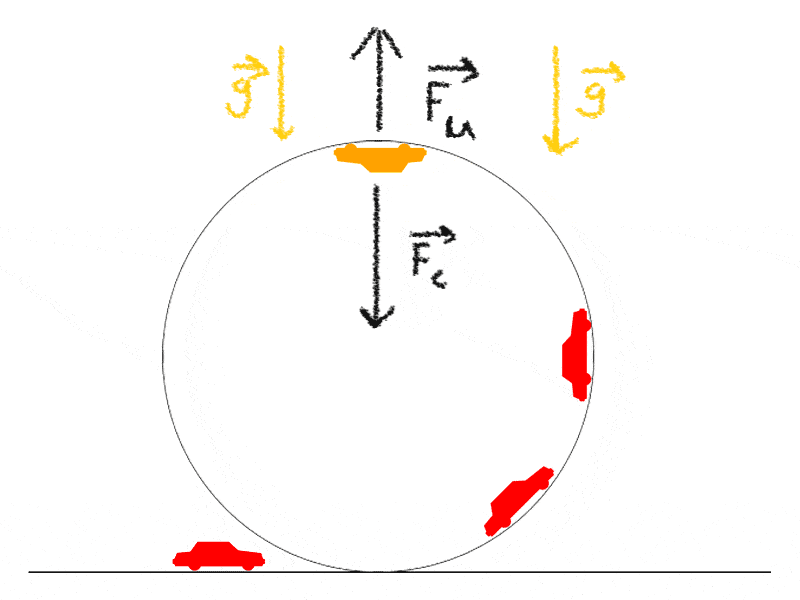# Centrifugal/Centripetal forceIn the figure, at the point where the orange car is at, what is preventing the car from falling down to the earth? Where does the force $$\vec{F}_u$$ comes from?

From the car's velocity? If so, how could it? Isn't the velocity at the point completely in the x direction? Thus, there is no y direction to counteract the pull of gravity and centripetal force.

In this case, is the centripetal force created by the car's weight and the "normal force"? By normal force, i mean that the car drives into the loop-d-loop and that creates the normal force. Also, is there even a normal force when the car is at the point where the orange car is?

sorry :( i find this really confusing. I want to say the centrifugal "force" is creating upward force necessary to keep car from falling, but where does it come from? The force of the weight is provided by the earth, the centripetal force is provided by...???

Philip Wood
Gold Member
The car is falling. Just as a stone is falling when you throw it horizontally. It's moving so fast horizontally at the same time that you might not notice it falling. (In fact the flattish top of the parabola is, to second order, a circle, and if you pursue this argument, the familiar g =v^2/r formula emerges.)

(In the case of the car, if moving very fast, the drum is probably forcing it fall (as it follows the curve) faster than it would fall if there were no drum.)

Last edited:
Doc Al
Mentor
In the figure, at the point where the orange car is at, what is preventing the car from falling down to the earth? Where does the force $$\vec{F}_u$$ comes from?
There's no such force. All forces acting on the car at that point act downward.

From the car's velocity? If so, how could it? Isn't the velocity at the point completely in the x direction? Thus, there is no y direction to counteract the pull of gravity and centripetal force.
What matters is the direction of the car's acceleration, which is downward. To maintain the circular motion, the car requires a net downward force.

In this case, is the centripetal force created by the car's weight and the "normal force"? By normal force, i mean that the car drives into the loop-d-loop and that creates the normal force. Also, is there even a normal force when the car is at the point where the orange car is?
Sure, as long as the car is moving fast enough.

sorry :( i find this really confusing. I want to say the centrifugal "force" is creating upward force necessary to keep car from falling, but where does it come from? The force of the weight is provided by the earth, the centripetal force is provided by...???
Forget about centrifugal force (that's only useful when describing things from a rotating frame). Gravity and the normal force provide the centripetal force.

rcgldr
Homework Helper
It may be easier to understand this by looking at the acceleration involved. At the top of the loop, assuming the car is moving fast enough and the radius of the path is small enough (v2 / r >= 1 g), then the moving point of contact between car and looped track is accelerating downwards at or greater than 1 g, so the car remains in contact with the track because the point of contact is accelerating downwards faster than gravity is accelerating the car away from the point of contact.

I would reinforce Doc Al's statement . There is no Fu. There are only 2 forces acting on the car
1)gravity
2)the (normal) reaction of the track
The resultant force has a value of mv^2/r or mω^2r